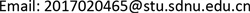﻿ 耦合二阶ODE波系统的输出反馈镇定 Output Feedback Stabilization of a Coupled Second Order ODE-Wave System

Vol. 07  No. 12 ( 2018 ), Article ID: 27817 , 9 pages
10.12677/AAM.2018.712171

Output Feedback Stabilization of a Coupled Second Order ODE-Wave System

Liping Wang, Fengfei Jin

School of Mathematics and Statistics, Shandong Normal University, Jinan ShandongReceived: Nov. 1st, 2018; accepted: Nov. 23rd, 2018; published: Nov. 30th, 2018ABSTRACT

In this paper, we are concerned with boundary output feedback stabilization of a coupled second order ODE-wave system. We first design an observer for ODE-wave system by output of original system and the effectiveness of observer is proved. Then an output feedback controller is proposed based on a state feedback controller in  . Operator semi-group method and back-stepping transformation are adopted to prove that the resulting closed-loop system admits a unique solution in state space and the solution of closed-loop system is asymptotically stable.

Keywords:Output Feedback, Back-Stepping, Stabilization, Controller Design1. 引言

$\left\{\begin{array}{l}{y}^{″}\left(t\right)={a}^{2}y\left(t\right)+bu\left({x}_{0},t\right),t>0,\\ {u}_{tt}\left(x,t\right)={u}_{xx}\left(x,t\right),x\in \left(0,1\right),t>0,\\ u\left(0,t\right)=0,{u}_{x}\left(1,t\right)=U\left(t\right),t\ge 0,\\ {y}_{out}\left(t\right)=\left\{y\left(t\right),{u}_{t}\left(1,t\right)\right\},t\ge 0,\\ y\left(0\right)={y}_{0},{y}^{\prime }\left(0\right)={y}_{1},\\ u\left(x,0\right)={u}_{0}\left(x\right),{u}_{t}\left(x,0\right)={u}_{1}\left(x\right),x\in \left(0,1\right),\end{array}$ (1.1)

$\left\{\begin{array}{l}\stackrel{˙}{X}\left(t\right)=AX\left(t\right)+Bu\left({x}_{0},t\right),t>0,\\ {u}_{tt}\left(x,t\right)={u}_{xx}\left(x,t\right),x\in \left(0,1\right),t>0,\\ u\left(0,t\right)=0,{u}_{x}\left(1,t\right)=U\left(t\right),t\ge 0,\\ {y}_{out}\left(t\right)=\left\{{x}_{1}\left(t\right),{u}_{t}\left(1,t\right)\right\},t\ge 0,\\ X\left(0\right)={X}_{0},u\left(x,0\right)={u}_{0}\left(x\right),{u}_{t}\left(x,0\right)={u}_{1}\left(x\right),x\in \left(0,1\right),\end{array}$ (1.2)

$A=\left(\begin{array}{l}0\text{}1\\ {a}^{2}\text{}0\end{array}\right),B=\left(\begin{array}{l}0\\ b\end{array}\right),{X}_{0}=\left(\begin{array}{l}{y}_{0}\\ {y}_{1}\end{array}\right).$

2. 观测器和控制器设计

$\left\{\begin{array}{l}{\stackrel{˙}{\stackrel{^}{x}}}_{1}\left(t\right)={\stackrel{^}{x}}_{2}\left(t\right)+{q}_{1}\left({\stackrel{^}{x}}_{1}\left(t\right)-{x}_{1}\left(t\right)\right),t>0,\\ {\stackrel{˙}{\stackrel{^}{x}}}_{2}\left(t\right)={a}^{2}{\stackrel{^}{x}}_{1}\left(t\right)+{q}_{2}\left({\stackrel{^}{x}}_{1}\left(t\right)-{x}_{1}\left(t\right)\right)+b\stackrel{^}{u}\left({x}_{0},t\right),t>0,\\ {\stackrel{^}{u}}_{tt}\left(x,t\right)={\stackrel{^}{u}}_{xx}\left(x,t\right),t>0,\\ \stackrel{^}{u}\left(0,t\right)=0,t\ge 0,\\ {\stackrel{^}{u}}_{x}\left(1,t\right)=U\left(t\right)-k\left({\stackrel{^}{u}}_{t}\left(1,t\right)-{u}_{t}\left(1,t\right)\right),t\ge 0,\\ {\stackrel{^}{x}}_{1}\left(0\right)={\stackrel{^}{x}}_{10},{\stackrel{^}{x}}_{2}\left(0\right)={\stackrel{^}{x}}_{20},\\ \stackrel{^}{u}\left(x,0\right)={\stackrel{^}{u}}_{0}\left(x\right),{\stackrel{^}{u}}_{t}\left(x,0\right)={\stackrel{^}{u}}_{1}\left(x\right),\end{array}$ (2.1)

$\left\{\begin{array}{l}{\stackrel{˙}{\stackrel{˜}{x}}}_{1}\left(t\right)={\stackrel{˜}{x}}_{2}\left(t\right)+{q}_{1}{\stackrel{˜}{x}}_{1}\left(t\right),t>0,\\ {\stackrel{˙}{\stackrel{˜}{x}}}_{2}\left(t\right)={a}^{2}{\stackrel{˜}{x}}_{1}\left(t\right)+{q}_{2}{\stackrel{˜}{x}}_{1}\left(t\right)+b\stackrel{˜}{u}\left({x}_{0},t\right),t>0,\\ {\stackrel{˜}{u}}_{tt}\left(x,t\right)={\stackrel{˜}{u}}_{xx}\left(x,t\right),t>0,\\ \stackrel{˜}{u}\left(0,t\right)=0,t\ge 0,\\ {\stackrel{˜}{u}}_{x}\left(1,t\right)=-k{\stackrel{˜}{u}}_{t}\left(1,t\right),t\ge 0,\\ {\stackrel{˜}{x}}_{1}\left(0\right)={\stackrel{^}{x}}_{10}-{x}_{10},{\stackrel{˜}{x}}_{2}\left(0\right)={\stackrel{^}{x}}_{20}-{x}_{20},\\ \stackrel{˜}{u}\left(x,0\right)={\stackrel{^}{u}}_{0}\left(x\right)-{u}_{0}\left(x\right),{\stackrel{˜}{u}}_{t}\left(x,0\right)={\stackrel{˜}{u}}_{1}\left(x\right)-{u}_{1}\left(x\right).\end{array}$ (2.2)

$Η=\left\{{\left(f,g\right)}^{Τ}\in {H}^{1}\left(0,1\right)×{L}^{2}\left(0,1\right)|f\left(0\right)=0\right\}$

${‖{\left(f,g\right)}^{Τ}‖}_{Η}^{2}={\int }_{0}^{1}\left({|{f}^{\prime }\left(x\right)|}^{2}+{|g\left(x\right)|}^{2}\right)\text{d}x.$

${‖{\left(\stackrel{˜}{u}\left(\cdot ,t\right),{\stackrel{˜}{u}}_{t}\left(\cdot ,t\right)\right)}^{Τ}‖}_{Η}\le M{\text{e}}^{-\delta t}{‖{\left(\stackrel{˜}{u}\left(\cdot ,0\right),{\stackrel{˜}{u}}_{t}\left(\cdot ,0\right)\right)}^{Τ}‖}_{Η}.$ (2.3)

$|\stackrel{˜}{u}\left({x}_{0},t\right)|\le {‖{\left(\stackrel{˜}{u}\left(\cdot ,t\right),{\stackrel{˜}{u}}_{t}\left(\cdot ,t\right)\right)}^{Τ}‖}_{Η}\le M{\text{e}}^{-\delta t}{‖{\left(\stackrel{˜}{u}\left(\cdot ,0\right),{\stackrel{˜}{u}}_{t}\left(\cdot ,0\right)\right)}^{Τ}‖}_{Η}.$ (2.4)

${\left({\stackrel{˜}{x}}_{1},{\stackrel{˜}{x}}_{2}\right)}^{Τ}={\text{e}}^{{A}_{1}t}{\left({\stackrel{˜}{x}}_{1}\left(0\right),{\stackrel{˜}{x}}_{2}\left(0\right)\right)}^{Τ}+{\int }_{0}^{t}{\text{e}}^{{A}_{1}\left(t-s\right)}B\stackrel{˜}{u}\left({x}_{0},s\right)\text{d}s,$

${A}_{1}=\left(\begin{array}{l}\text{}{q}_{1}\text{}1\\ {a}^{2}+{q}_{2}\text{}0\end{array}\right),$ (2.5)

${q}_{1}<0$${q}_{2}<-{a}^{2}$ 是可调常数。很明显，对于这样选择的参数， ${A}_{1}$ 是Hurwitz的。从而 ${\left({\stackrel{˜}{x}}_{1},{\stackrel{˜}{x}}_{2}\right)}^{Τ}$ 是指数稳定的。因此我们有如下引理：

$\begin{array}{l}U\left(t\right)={\int }_{0}^{1}\left[c{l}_{yy}\left(1,y\right)+{k}_{x}\left(1,y\right)\right]\stackrel{^}{u}\left(y,t\right)\text{d}y-c{l}_{y}\left(1,1\right)\stackrel{^}{u}\left(1,t\right)\\ \text{}+{\int }_{0}^{{x}_{0}}\left[{{p}^{\prime }}_{1}\left(1\right){q}_{1}\left(y\right)+cp\left(1\right)q\left(y\right)\right]{\stackrel{^}{u}}_{t}\left(y,t\right)\text{d}y+{\varphi }^{\prime }\left(1\right)\stackrel{^}{X}\left(t\right)\\ \text{}+{\int }_{0}^{{x}_{0}}\left[{p}^{\prime }\left(1\right)q\left(y\right)+c{p}_{1}\left(1\right){{q}^{″}}_{1}\left(y\right)\right]\stackrel{^}{u}\left(y,t\right)\text{d}y+c\varphi \left(1\right)A\stackrel{^}{X}\left(t\right)\\ \text{}+{\int }_{0}^{1}\left[{l}_{x}\left(1,y\right)+ck\left(1,y\right)\right]{\stackrel{^}{u}}_{t}\left(y,t\right)\text{d}y+c{u}_{t}\left(1,t\right),\end{array}$ (2.6)

$\left\{\begin{array}{l}k\left(x,y\right)=-\xi \eta \left(1-{\text{e}}^{2\lambda {x}_{0}}\right)\left({\text{e}}^{\lambda \left(x-y\right)}-{\text{e}}^{-\lambda \left(x-y\right)}\right)\right),\\ l\left(x,y\right)=-{\xi }_{1}{\eta }_{1}\left(1-{\text{e}}^{2{\lambda }_{1}{x}_{0}}\right)\left({\text{e}}^{{\lambda }_{1}\left(x-y\right)}-{\text{e}}^{-{\lambda }_{1}\left(x-y\right)}\right),\\ p\left(x\right)=\xi {\text{e}}^{\lambda x}-\xi {\text{e}}^{-\lambda x},\\ q\left(y\right)=\eta {\text{e}}^{\lambda y}-\eta {\text{e}}^{-\lambda \left(2{x}_{0}-y\right)},\\ {p}_{1}\left(x\right)={\xi }_{1}{\text{e}}^{{\lambda }_{1}x}-{\xi }_{1}{\text{e}}^{-{\lambda }_{1}x},\\ {q}_{1}\left(y\right)={\eta }_{1}{\text{e}}^{{\lambda }_{1}y}-{\eta }_{1}{\text{e}}^{-{\lambda }_{1}\left(2{x}_{0}-y\right)},\\ \varphi \left(x\right)=\frac{{\text{e}}^{{\lambda }_{1}x}-{\text{e}}^{-{\lambda }_{1}x}}{{\text{e}}^{{\lambda }_{1}{x}_{0}}-{\text{e}}^{-{\lambda }_{1}{x}_{0}}}K,\\ \xi \eta =\frac{KAB}{2\lambda {\text{e}}^{\lambda {x}_{0}}\left({\text{e}}^{\lambda {x}_{0}}-{\text{e}}^{-\lambda {x}_{0}}\right)},\\ {\xi }_{1}{\eta }_{1}=\frac{KB}{2{\lambda }_{1}{\text{e}}^{{\lambda }_{1}{x}_{0}}\left({\text{e}}^{{\lambda }_{1}{x}_{0}}-{\text{e}}^{-{\lambda }_{1}{x}_{0}}\right)}\\ \lambda ={\lambda }_{1}=a.\end{array}$ (2.7)

$\left\{\begin{array}{l}\stackrel{˙}{X}\left(t\right)=AX\left(t\right)+Bu\left({x}_{0},t\right),t>0,\\ {u}_{tt}\left(x,t\right)={u}_{xx}\left(x,t\right),x\in \left(0,1\right),t>0,\\ u\left(0,t\right)=0,t>0,\\ {u}_{x}\left(1,t\right)={\int }_{0}^{1}\left[c{l}_{yy}\left(1,y\right)+{k}_{x}\left(1,y\right)\right]\stackrel{^}{u}\left(y,t\right)\text{d}y-c{l}_{y}\left(1,1\right)\stackrel{^}{u}\left(1,t\right)\\ \text{}+{\int }_{0}^{{x}_{0}}\left[{{p}^{\prime }}_{1}\left(1\right){q}_{1}\left(y\right)+cp\left(1\right)q\left(y\right)\right]{\stackrel{^}{u}}_{t}\left(y,t\right)\text{d}y+{\varphi }^{\prime }\left(1\right)\stackrel{^}{X}\left(t\right)\\ \text{}+{\int }_{0}^{{x}_{0}}\left[{p}^{\prime }\left(1\right)q\left(y\right)+c{p}_{1}\left(1\right){{q}^{″}}_{1}\left(y\right)\right]\stackrel{^}{u}\left(y,t\right)\text{d}y+c\varphi \left(1\right)A\stackrel{^}{X}\left(t\right)\\ \text{}+{\int }_{0}^{1}\left[{l}_{x}\left(1,y\right)+ck\left(1,y\right)\right]{\stackrel{^}{u}}_{t}\left(y,t\right)\text{d}y+c{u}_{t}\left(1,t\right),t>0,\\ {\stackrel{˙}{\stackrel{^}{x}}}_{1}\left(t\right)={\stackrel{^}{x}}_{2}\left(t\right)+{q}_{1}\left({\stackrel{^}{x}}_{1}\left(t\right)-{x}_{1}\left(t\right)\right),t>0,\\ {\stackrel{˙}{\stackrel{^}{x}}}_{2}\left(t\right)={a}^{2}{\stackrel{^}{x}}_{1}\left(t\right)+{q}_{2}\left({\stackrel{^}{x}}_{1}\left(t\right)-{x}_{1}\left(t\right)\right)+b\stackrel{^}{u}\left({x}_{0},t\right),t>0,\\ {\stackrel{^}{u}}_{tt}\left(x,t\right)={\stackrel{^}{u}}_{xx}\left(x,t\right),t>0,\\ \stackrel{^}{u}\left(0,t\right)=0,t\ge 0,\\ {\stackrel{^}{u}}_{x}\left(1,t\right)={u}_{x}\left(1,t\right)-k\left({\stackrel{^}{u}}_{t}\left(1,t\right)-{u}_{t}\left(1,t\right)\right),t\ge 0,\end{array}$ (2.8)

3. 闭环系统适定性与稳定性

$\left\{\begin{array}{l}\stackrel{˙}{X}\left(t\right)=AX\left(t\right)+Bu\left({x}_{0},t\right),t>0,\\ {u}_{tt}\left(x,t\right)={u}_{xx}\left(x,t\right),x\in \left(0,1\right),t>0,\\ u\left(0,t\right)=0,t>0,\\ {u}_{x}\left(1,t\right)={\int }_{0}^{1}\left[c{l}_{yy}\left(1,y\right)+{k}_{x}\left(1,y\right)\right]u\left(y,t\right)\text{d}y-c{l}_{y}\left(1,1\right)u\left(1,t\right)\\ \text{}+{\int }_{0}^{{x}_{0}}\left[{{p}^{\prime }}_{1}\left(1\right){q}_{1}\left(y\right)+cp\left(1\right)q\left(y\right)\right]{u}_{t}\left(y,t\right)\text{d}y+{\varphi }^{\prime }\left(1\right)X\left(t\right)\\ \text{}+{\int }_{0}^{{x}_{0}}\left[{p}^{\prime }\left(1\right)q\left(y\right)+c{p}_{1}\left(1\right){{q}^{″}}_{1}\left(y\right)\right]u\left(y,t\right)\text{d}y+c\varphi \left(1\right)AX\left(t\right)\\ \text{}+{\int }_{0}^{1}\left[{l}_{x}\left(1,y\right)+ck\left(1,y\right)\right]{u}_{t}\left(y,t\right)\text{d}y+c{u}_{t}\left(1,t\right)\\ \text{}+{\int }_{0}^{1}\left[c{l}_{yy}\left(1,y\right)+{k}_{x}\left(1,y\right)\right]\stackrel{˜}{u}\left(y,t\right)\text{d}y-c{l}_{y}\left(1,1\right)\stackrel{˜}{u}\left(1,t\right)\\ \text{}+{\int }_{0}^{{x}_{0}}\left[{{p}^{\prime }}_{1}\left(1\right){q}_{1}\left(y\right)+cp\left(1\right)q\left(y\right)\right]{\stackrel{˜}{u}}_{t}\left(y,t\right)\text{d}y+{\varphi }^{\prime }\left(1\right)\stackrel{˜}{X}\left(t\right)\\ \text{}+{\int }_{0}^{{x}_{0}}\left[{p}^{\prime }\left(1\right)q\left(y\right)+c{p}_{1}\left(1\right){{q}^{″}}_{1}\left(y\right)\right]\stackrel{˜}{u}\left(y,t\right)\text{d}y+c\varphi \left(1\right)A\stackrel{˜}{X}\left(t\right)\\ \text{}+{\int }_{0}^{1}\left[{l}_{x}\left(1,y\right)+ck\left(1,y\right)\right]{\stackrel{˜}{u}}_{t}\left(y,t\right)\text{d}y,t>0,\\ {\stackrel{˙}{\stackrel{˜}{x}}}_{1}\left(t\right)={\stackrel{˜}{x}}_{2}\left(t\right)+{q}_{1}{\stackrel{˜}{x}}_{1}\left(t\right),t>0,\\ {\stackrel{˙}{\stackrel{˜}{x}}}_{2}\left(t\right)={a}^{2}{\stackrel{˜}{x}}_{1}\left(t\right)+{q}_{2}{\stackrel{˜}{x}}_{1}\left(t\right)+b\stackrel{˜}{u}\left({x}_{0},t\right),t>0,\\ {\stackrel{˜}{u}}_{tt}\left(x,t\right)={\stackrel{˜}{u}}_{xx}\left(x,t\right),t>0,\\ \stackrel{˜}{u}\left(0,t\right)=0,t\ge 0,\\ {\stackrel{˜}{u}}_{x}\left(1,t\right)=-k{\stackrel{˜}{u}}_{t}\left(1,t\right),t\ge 0.\end{array}$ (3.1)

$\begin{array}{c}w\left(x,t\right)=u\left(x,t\right)-{\int }_{0}^{x}k\left(x,y\right)u\left(y,t\right)\text{d}y-{\int }_{0}^{x}l\left(x,y\right){u}_{t}\left(y,t\right)\text{d}y\\ \text{\hspace{0.17em}}\text{\hspace{0.17em}}-p\left(x\right){\int }_{0}^{{x}_{0}}q\left(y\right)u\left(y,t\right)\text{d}y-{p}_{1}\left(x\right){\int }_{0}^{{x}_{0}}{q}_{1}\left(y\right){u}_{t}\left(y,t\right)\text{d}y-\varphi \left(x\right)X\left(x\right).\end{array}$ (3.2)

$\left\{\begin{array}{l}\stackrel{˙}{X}\left(t\right)=\left(A+BK\right)X\left(t\right)+Bw\left({x}_{0},t\right),t>0,\\ {w}_{tt}\left(x,t\right)={w}_{xx}\left(x,t\right),x\in \left(0,1\right),t>0,\\ w\left(0,t\right)=0,t>0,\\ {w}_{x}\left(1,t\right)=-c{w}_{t}\left(1,t\right)+f\left(t\right),t>0,\\ w\left(x,0\right)={w}_{0}\left(x\right),{w}_{t}\left(x,0\right)={w}_{1}\left(x\right),x\in \left(0,1\right),\\ X\left(0\right)={X}_{0},\end{array}$ (3.3)

$\begin{array}{c}f\left(t\right)={\int }_{0}^{1}\left[c{l}_{yy}\left(1,y\right)+{k}_{x}\left(1,y\right)\right]\stackrel{˜}{u}\left(y,t\right)\text{d}y-c{l}_{y}\left(1,1\right)\stackrel{˜}{u}\left(1,t\right)\\ \text{\hspace{0.17em}}\text{\hspace{0.17em}}+{\int }_{0}^{{x}_{0}}\left[{{p}^{\prime }}_{1}\left(1\right){q}_{1}\left(y\right)+cp\left(1\right)q\left(y\right)\right]{\stackrel{˜}{u}}_{t}\left(y,t\right)\text{d}y+{\varphi }^{\prime }\left(1\right)\stackrel{˜}{X}\left(t\right)\\ \text{\hspace{0.17em}}\text{\hspace{0.17em}}+{\int }_{0}^{{x}_{0}}\left[{p}^{\prime }\left(1\right)q\left(y\right)+c{p}_{1}\left(1\right){{q}^{″}}_{1}\left(y\right)\right]\stackrel{˜}{u}\left(y,t\right)\text{d}y+c\varphi \left(1\right)A\stackrel{˜}{X}\left(t\right)\\ \text{\hspace{0.17em}}\text{\hspace{0.17em}}+{\int }_{0}^{1}\left[{l}_{x}\left(1,y\right)+ck\left(1,y\right)\right]{\stackrel{˜}{u}}_{t}\left(y,t\right)\text{d}y.\end{array}$ (3.4)

$|f\left(t\right)|\le {M}_{1}{\text{e}}^{-{\delta }_{1}t},t\ge 0.$

$\left\{\begin{array}{l}{w}_{tt}\left(x,t\right)={w}_{xx}\left(x,t\right),x\in \left(0,1\right),t>0,\\ w\left(0,t\right)=0,t>0,\\ {w}_{x}\left(1,t\right)=-c{w}_{t}\left(1,t\right)+f\left(t\right),t>0,\\ w\left(x,0\right)={w}_{0}\left(x\right),{w}_{t}\left(x,0\right)={w}_{1}\left(x\right),x\in \left(0,1\right),\\ X\left(0\right)={X}_{0}.\end{array}$ (3.5)

$\left\{\begin{array}{l}Α{\left(f,g\right)}^{Τ}={\left(g,{f}^{″}\right)}^{Τ},\forall {\left(f,g\right)}^{Τ}\in D\left(Α\right),\\ D\left(Α\right)=\left\{{\left(f,g\right)}^{Τ}\in {H}^{2}\left(0,1\right)×{H}^{1}\left(0,1\right)|Α{\left(f,g\right)}^{Τ}\in Η,{f}^{\prime }\left(1\right)=-cg\left(1\right)\right\}.\end{array}$ (3.6)

$\left\{\begin{array}{l}{\text{A}}^{\ast }\left(\begin{array}{l}\varphi \\ \psi \end{array}\right)=\left(\begin{array}{l}-{\psi }_{1}\\ -{\varphi }^{″}\end{array}\right),\forall \left(\begin{array}{l}\varphi \\ \psi \end{array}\right)\in D\left({\text{A}}^{\ast }\right),\\ D\left({\text{A}}^{\ast }\right)=\left\{{\left(\varphi ,\psi \right)}^{Τ}\in {H}^{2}\left(0,1\right)×{H}^{1}\left(0,1\right)|{\text{A}}^{\ast }\left(\varphi ,\psi \right)\in \text{H},{\varphi }^{\prime }\left(1\right)=c\psi \left(1\right)\right\}.\end{array}$ (3.7)

$\frac{\text{d}}{\text{d}t}〈\left(\begin{array}{l}w\\ {w}_{t}\end{array}\right),\left(\begin{array}{l}\varphi \\ \psi \end{array}\right)〉=〈\left(\begin{array}{l}w\\ {w}_{t}\end{array}\right),{Α}^{\ast }\left(\begin{array}{l}\varphi \\ \psi \end{array}\right)〉+〈\left(\begin{array}{l}\text{}0\\ \delta \left(x-1\right)\end{array}\right)f\left(t\right),\left(\begin{array}{l}\varphi \\ \psi \end{array}\right)〉,$ (3.8)

$\frac{\text{d}}{\text{d}t}{\left(w\left(\cdot ,t\right),{w}_{t}\left(\cdot ,t\right)\right)}^{Τ}=Α{\left(w\left(\cdot ,t\right),{w}_{t}\left(\cdot ,t\right)\right)}^{Τ}+Βf\left(t\right),$ (3.9)

$‖{\text{e}}^{Αt}‖\le K{\text{e}}^{-\mu t},t\ge 0.$ (3.10)

$\left\{\begin{array}{l}{w}_{tt}^{\ast }\left(x,t\right)={w}_{xx}^{\ast }\left(x,t\right),x\in \left(0,1\right),t>0,\\ {w}^{\ast }\left(0,t\right)=0,t>0,\\ {w}_{x}^{\ast }\left(1,t\right)=-c{w}_{t}^{\ast }\left(1,t\right),t>0,\\ {y}_{0}\left(t\right)=-{w}_{t}^{\ast }\left(1,t\right),t\ge 0.\end{array}$ (3.11)

${E}^{\ast }\left(t\right)=\frac{1}{2}{\int }_{0}^{1}\left({w}_{t}^{\ast 2}\left(x,t\right)+{w}_{x}^{\ast 2}\left(x,t\right)\right)\text{d}x.$

$E\left(t\right)$ 沿系统(3.11)的解对t求导得到

$\stackrel{˙}{E}\left(t\right)=-c{\left[{w}_{t}^{\ast }\left(1,t\right)\right]}^{2}.$ (3.12)

${\int }_{0}^{T}{\left[{w}_{t}^{\ast }\left(1,t\right)\right]}^{2}\text{d}t=\frac{1}{c}\left(E\left(0\right)-E\left(T\right)\right)\le \frac{1}{c}E\left(0\right).$

$\left\{\begin{array}{l}{Α}^{\ast -1}\left(\begin{array}{l}\varphi \\ \psi \end{array}\right)=\left(\begin{array}{c}-cx\varphi \left(1\right)+{\int }_{0}^{x}{\int }_{s}^{1}\psi \left(t\right)\text{d}t\text{d}s\\ \varphi \end{array}\right)，\\ {Β}^{\ast }{Α}^{\ast -1}\left(\begin{array}{l}\varphi \\ \psi \end{array}\right)=\left(\begin{array}{c}0\\ -\varphi \left(1\right)\end{array}\right),\forall {\left(\varphi ,\psi \right)}^{\prime }\in Η.\text{}\end{array}$ (3.13)

${|-\phi \left(1\right)|}^{2}={\phi }^{2}\left(1\right)\le {\int }_{0}^{1}{{\phi }^{\prime }}^{2}\left(x\right)\text{d}x\le {‖\left(\phi ,\psi \right)‖}_{Η}^{2}.$ (3.14)

(3.5)的解可以写成(  )

${\left(w\left(\cdot ,t\right),{w}_{t}\left(\cdot ,t\right)\right)}^{Τ}={\text{e}}^{Αt}{\left({w}_{0},{w}_{1}\right)}^{Τ}+{\int }_{0}^{t}{\text{e}}^{Α\left(t-s\right)}Βf\left(s\right)\text{d}s.$

${‖{\left(w\left(\cdot ,t\right),{w}_{t}\left(\cdot ,t\right)\right)}^{Τ}‖}_{\text{H}}\le {M}_{2}{\text{e}}^{-{\delta }_{2}t}{‖{\left(w\left(\cdot ,0\right),{w}_{t}\left(\cdot ,0\right)\right)}^{Τ}‖}_{\text{H}},t\ge 0.$ (3.15)

$|w\left({x}_{0},t\right)|\le {‖{\left(w\left(\cdot ,t\right),{w}_{t}\left(\cdot ,t\right)\right)}^{Τ}‖}_{Η}\le {M}_{2}{\text{e}}^{-{\delta }_{2}t}{‖{\left(w\left(\cdot ,0\right),{w}_{t}\left(\cdot ,0\right)\right)}^{Τ}‖}_{Η}.$

$X\left(t\right)={\text{e}}^{\left(A+BK\right)t}{X}_{0}+{\int }_{0}^{t}{\text{e}}^{\left(A+BK\right)\left(t-s\right)}Bw\left({x}_{0},s\right)\text{d}s,$ (3.16)

$\begin{array}{c}u\left(x,t\right)=w\left(x,t\right)-{\int }_{0}^{x}n\left(x,y\right)w\left(y,t\right)\text{d}y-{\int }_{0}^{x}m\left(x,y\right){w}_{t}\left(y,t\right)\text{d}y\\ \text{\hspace{0.17em}}\text{\hspace{0.17em}}-g\left(x\right){\int }_{0}^{{x}_{0}}h\left(y\right)w\left(y,t\right)\text{d}y-{g}_{1}\left(x\right){\int }_{0}^{{x}_{0}}{h}_{1}\left(y\right){w}_{t}\left(y,t\right)\text{d}y-\psi \left(x\right)X\left(t\right)，\end{array}$ (3.17)

$\left\{\begin{array}{l}{n}_{xx}\left(x,y\right)-{n}_{yy}\left(x,y\right)=0,\\ n\left(x,x\right)=0,n\left(x,0\right)=-g\left(x\right)h\left(0\right),\\ {m}_{xx}\left(x,y\right)-{m}_{yy}\left(x,y\right)=0,\\ m\left(x,x\right)=0,m\left(x,0\right)=-{g}_{1}\left(x\right){h}_{1}\left(0\right),\\ {g}^{″}\left(x\right)h\left(y\right)-g\left(x\right){h}^{″}\left(y\right)=0,\\ h\left({x}_{0}\right)=0,g\left(x\right){h}^{\prime }\left({x}_{0}\right)=\psi \left(x\right)\left(A+BK\right)B,\\ g\left(0\right)=0,n\left({x}_{0},y\right)+g\left(x\right)h\left(y\right)=0,\\ {{g}^{″}}_{1}\left(x\right){h}_{1}\left(y\right)-{g}_{1}\left(x\right){{h}^{″}}_{1}\left(y\right)=0,\\ {g}_{1}\left({x}_{0}\right)=0,{g}_{1}\left(x\right){{h}^{\prime }}_{1}\left({x}_{0}\right)=\psi \left(x\right)B,\\ {g}_{1}\left(0\right)=0,m\left({x}_{0},y\right)+{g}_{1}\left({x}_{0}\right){h}_{1}\left(y\right)=0,\\ {\psi }^{″}\left(x\right)-\psi \left(x\right){\left(A+BK\right)}^{2}=0,\\ \psi \left({x}_{0}\right)=K,\psi \left(0\right)=0,\end{array}$ (3.18)

${‖\left(X\left(t\right),u\left(\cdot ,t\right),{u}_{t}\left(\cdot ,t\right),\stackrel{^}{X}\left(t\right),\stackrel{^}{u}\left(\cdot ,t\right),{\stackrel{^}{u}}_{t}\left(\cdot ,t\right)\right)‖}_{{\left(\text{C}×Η\right)}^{2}}\le L{\text{e}}^{-\omega t}{‖\left({X}_{0},{u}_{0},{u}_{1},{\stackrel{^}{X}}_{0},{\stackrel{^}{u}}_{0},{\stackrel{^}{u}}_{1}\right)‖}_{{\left(\text{C}×Η\right)}^{2}},\forall t>0,$ (3.19)

Output Feedback Stabilization of a Coupled Second Order ODE-Wave System[J]. 应用数学进展, 2018, 07(12): 1477-1485. https://doi.org/10.12677/AAM.2018.712171

1. 1. Zhen, Z.Y., Wang, K., Zhou, Z.C. and Loxton, R. (2016) Stabilization of a Coupled Second Order ODE-Wave System. Proceedings of the 35th Chinese Control Conference, Chengdu, 27-29. https://doi.org/10.1109/ChiCC.2016.7553282

2. 2. Chen, G. (1979) Energy Decay Estimates and Exact Bounda-ry-Value Controllability for the Wave Equation in Abounded Domain. Journal de Mathematiques Pures et Appliquees, 58, 249-273.

3. 3. Krstic, M., Guo, B.Z., Blogh, A. and Smyshlyaev, A. (2008) Output Feedback Stabilization of an Unstable Wave Equation. Automatica, 44, 63-74. https://doi.org/10.1016/j.automatica.2007.05.012

4. 4. Smyshlyaev, A., Cerpa, E. and Krstic, M. (2010) Boundary Stabilization of a 1-D Wave Equation with In-Domain Anti-Damping. SIAM Journal on Control and Optimization, 48, 4014-4031. https://doi.org/10.1137/080742646

5. 5. d’Andr´ea-Novel, B. and Coron, J.M. (2000) Exponential Stabilization of an Overhead Crane with Flexible Cable via a Back-Stepping Approach. Automatica, 36, 587-593.

6. 6. Krstic, M. (2009) Compensating a String PDE in the Actuation or in Sensing Path of an Unstable ODE. IEEE Transaction on Automatic Control, 54, 1362-1368. https://doi.org/10.1109/TAC.2009.2015557

7. 7. Meglio, F.D., Argomedo, F.B., Gu, L. and Krstic, M. (2018) Stabilization of Coupled Linear Hetero Directional Hyperbolic PDE-ODE Systems. Automatica, 87, 281-289. https://doi.org/10.1016/j.automatica.2017.09.027

8. 8. Tang, S. and Xie, C. (2011) Stabilization for a Coupled PDE-ODE Control System. Journal of the Franklin Institute, 348, 2142-2155. https://doi.org/10.1016/j.jfranklin.2011.06.008

9. 9. Zhou, Z.Z. and Xu, C. (2015) Stabilization of a Second Order ODE-Heat System Coupling at Intermediate Point. Automatica, 60, 57-64. https://doi.org/10.1016/j.automatica.2015.06.039

10. 10. Weiss, G. (1989) Admissibility of Unbounded Control Operators. SIAM Journal on Control and Optimization, 27, 527-545. https://doi.org/10.1137/0327028

11. 11. Su, L., Guo, W., Wang, J. and Krstic, M. (2017) Boundary Stabilization of Wave Equation with Velocity Recirculation. IEEE Transactions on Automatic Control, 62, 4760-4767. https://doi.org/10.1109/TAC.2017.2688128